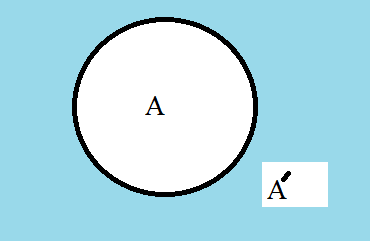## Latest:

latest

### Different set operations are done in different cases in Maths. For example, Union, Intersection, Minus, Universal, Complement, Family, and ...

Different set operations are done in different cases in Maths. For example, Union, Intersection, Minus, Universal, Complement, Family, and Power set.

## Different set operations in maths

So, below we have discussed these set operations sequentially.
• Union of sets
• Intersection of sets
• Minus of sets
• Universal set
• Complement of set
• Family of sets
• Power set

### Union of sets

If we build a set where all the elements of two sets will exist then that set will be called a union of sets.

#### Example

Let the sets are A = {1, 2, 3, 4} and B = {3, 4, 5, 6}. By taking all the elements from set A and set B a set can be built. That set is presented as A∪B[A union B].

A∪B = {1, 2, 3, 4, 5, 6} is the set. Mathematically A∪B = { x:x ∈ A or x ∈B}Union set of two sets in ven diagram

Remember: Don't repeat any element.

It can be said,
1. A∪B = B∪A
2. A⊂ A∪B and also B⊂ A∪B with respecting the definition of the subset.

### Intersection of sets

If we build a set where the common elements of two sets will exist then that set will be called the intersection of sets.

#### Example

Let the sets are A = {1, 2, 3, 4} and B = {3, 4, 5, 6}. By taking the common elements from set A and set B a set can be built. That set is presented as A∩B[A intersection B].

A∩B = {3, 4} is the set. Mathematically A∩B = { x:x ∈ A and x ∈ B}.Intersection set of two sets in ven diagram

Remember: If there is no common element between two sets then the intersection of those two sets will be an empty set.

It can be said,
1. (A∩B) ⊂ A and also (A∩B) ⊂ B with respecting the definition of the subset.

### Minus of sets

Minus of two sets is a set where the elements exist in the set but not in the set B. This set is represented as A-B(A minus B). Mathematically A-B = {x: x ∈ A , x∉B}Minus of two sets in ven diagram

#### Example

Let the sets are A = {1, 2, 3, 4} and B = {3, 4}. By taking the elements which are inset A but not in the set B we get,

A-B = {1,2} is the set. It can be said,
1. (A-B)⊂ A
2. Minus set of A and B is also represented with A\B.

### Universal set

In mathematics, the Universal set is the set that contains all objects or elements and of which all other sets are subsets. It is represented with U.Universal set in ven diagram

### Complement of set

If a set(A) is built with some of the elements of U(Universal set) then the set of rest of the elements of U is called the complement of set A concerning U. In easier words minus set of U and A is complement set of A.

The complement set of set A is represented with Aˊ. Aˊ = U-A.Complement set of set A in ven diagram

#### Example

Let N = {1, 2, 3, 4, 5, 6,........} is a set of all normal numbers and A = {1, 3, 5, 7, 9,......} is the set of all odd numbers.

then with respect to N complement set of A is
Aˊ = N-A
Aˊ = {2, 4, 6, 8,10, ........}

From union set, an intersection set knowledge we can say,

1. A∪Aˊ = U
2. A∩Aˊ = ∅

### Family of sets

If all the elements of a set are a set then the set is called the family of sets.
Example: A={{1},{1, 2},{2},{2, 3},{3}}

### Power set

If a family of sets is built by taking all the subsets from a set then that family of sets is called a power set. If a set is A then the power set of the set A will be P(A) [Power set of the set A].

#### Example

G = {1, 2, 3}
∴ P(G) = {{1, 2, 3},{1, 2},{1, 3},{2, 3},{1},{2},{3},∅}
If a set contains n number of elements then power set of that set will contain 2ⁿ numbers of elements.